# Your answer is partially correct. In the figure below, what is the potential difference Va Vc...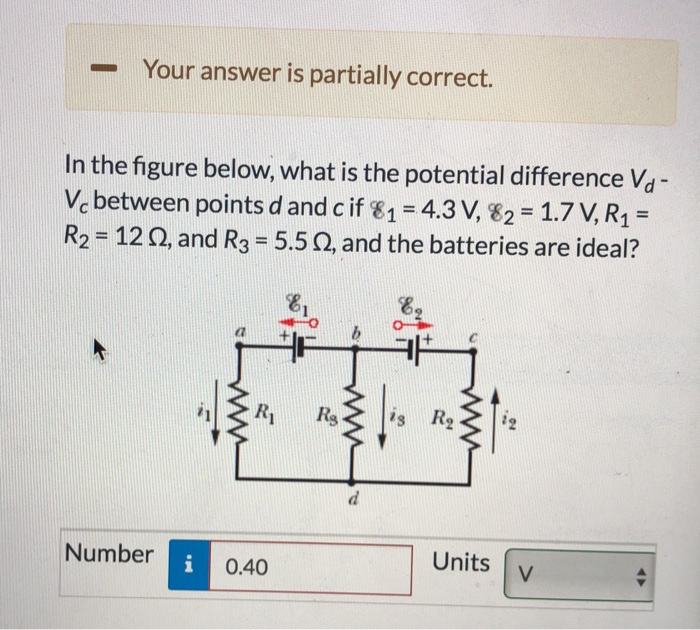Your answer is partially correct. In the figure below, what is the potential difference Va Vc between points d and cif 81-4.3 V, 82 1.7 V,R1- R2-12 0, and R3 5.50, and the batteries are ideal? Number Units v 0.40

We need at least 10 more requests to produce the answer.

0 / 10 have requested this problem solution

The more requests, the faster the answer.

All students who have requested the answer will be notified once they are available.

#### Earn Coin

Coins can be redeemed for fabulous gifts.

Similar Homework Help Questions
• ### Chapter 27, Problem 018 In the figure below, what is the potential difference Vd-V between points...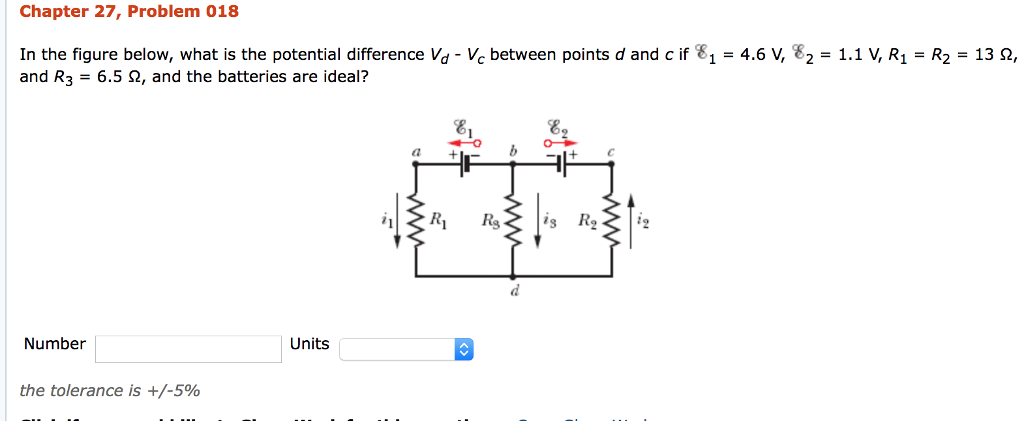Chapter 27, Problem 018 In the figure below, what is the potential difference Vd-V between points d and c if 81 = 4.6 V, 82 = 1.1 V, R1 = R2 = 13 ?, and R3-6.5 ?, and the batteries are ideal? F8 a + + C Number Units the tolerance is +/-590

• ### Chapter 27, Problem 023 Partially correct answer. Your answer is partially correct. Try again. In the...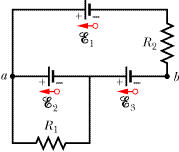Chapter 27, Problem 023 Partially correct answer. Your answer is partially correct. Try again. In the figure R1 = 130 Ω, R2 = 50 Ω, and the ideal batteries have emfs ε1 = 6.0 V, ε2 = 5.0 V, and ε3 = 4.0 V. Find (a) the current in R1, (b) the current in R2, and (c) the potential difference between points a and b. R2 8 R w

• ### Chapter 27, Problem 035 Your answer is partially correct. Try again. In the figure ε-15.0 V,...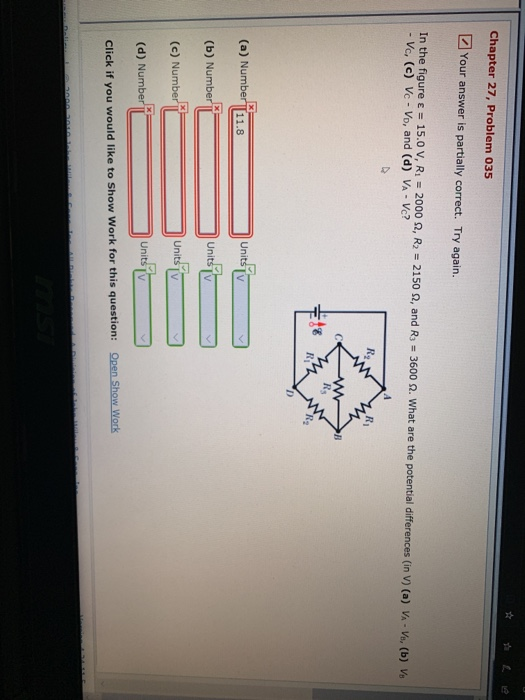Chapter 27, Problem 035 Your answer is partially correct. Try again. In the figure ε-15.0 V, Ri-2000S, R2-2150 Ω, and R3-3600 Ω. What are the potential differences (in V) (a) VA-V, (b) Vo - Vc, (c) Vc -Vo, and (d) VA- Vc? Rg (a) Number 11.8 (b) Number (c) Number (d) Number Click if you would like to Show Work for this question: units

• ### The circuit shown in the figure below contains three resistors (R1, R2, three batteries (VA, V8,...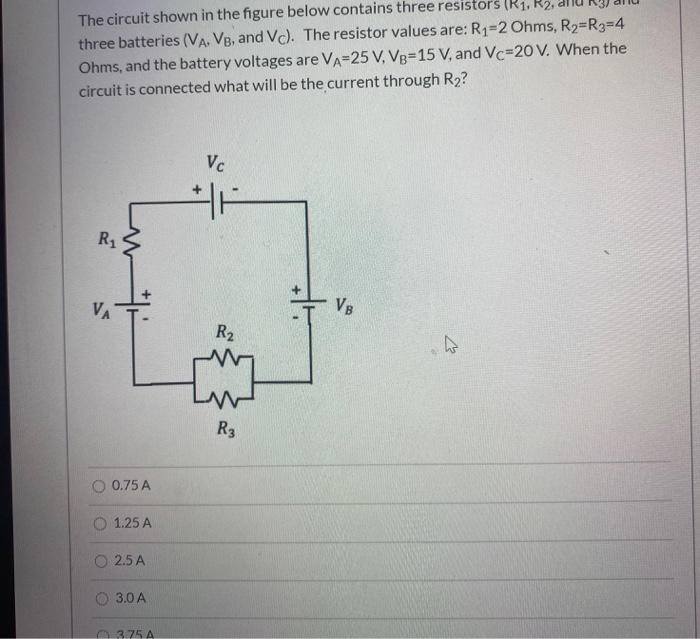The circuit shown in the figure below contains three resistors (R1, R2, three batteries (VA, V8, and Vc). The resistor values are: R1-2 Ohms, R2=R3=4 Ohms, and the battery voltages are Va=25 V, VB=15 V, and Vc=20 V. When the circuit is connected what will be the current through R2? VC R1 ** VA ++ VE R2 ho {} R3 0.75 A 1.25 A O 2.5 A O 3.0 A 375 A

• ### Chapter 27, Problem 032 Your answer is partially correct. Try again. Both batteries in Figure (a)...Chapter 27, Problem 032 Your answer is partially correct. Try again. Both batteries in Figure (a) are ideal. Emf 1 of battery 1 has a fixed value, but emf 2 of battery 2 can be varied between 35.0 V and 350.0 V. The plots in Figure (b) give the currents through the two batteries as a function of 12. The vertical scale is set by is = 0.48 A. You must decide which plot corresponds to which battery, but for...

• ### Find the potential difference between point a and point b for the situation shown below. Here...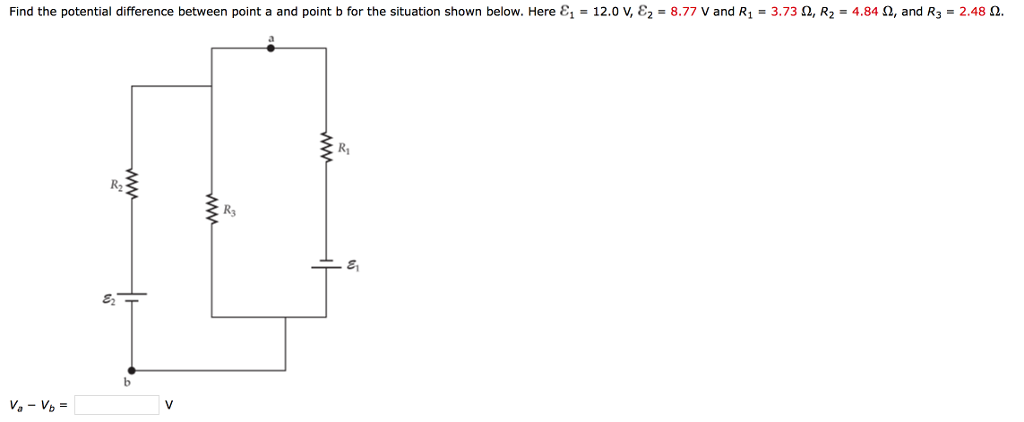Find the potential difference between point a and point b for the situation shown below. Here ?1-12.0 v, ?,-8.77 v and R1 = 3.73 ?, R2-4.84 ?, and R3-248 ?. Ri R2 R3 81 82

• ### The circuit shown in the figure below contains three resistors (R1, R2, and R3) and three...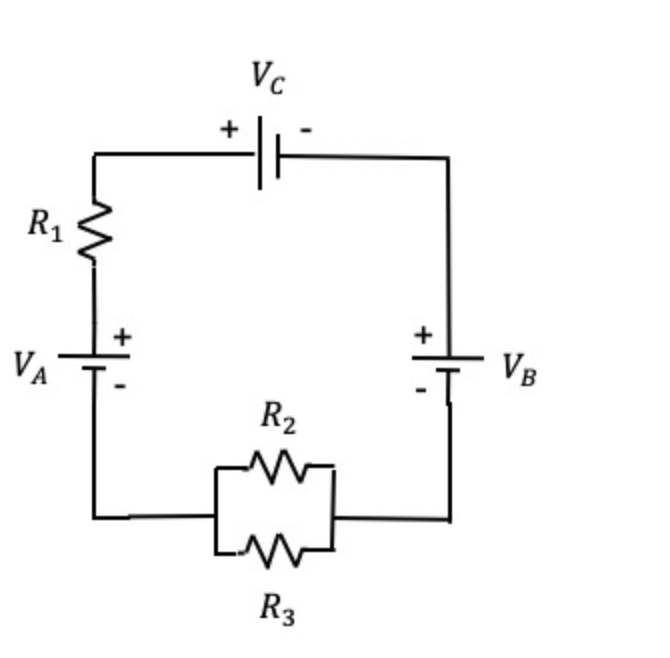The circuit shown in the figure below contains three resistors (R1, R2, and R3) and three batteries (VA, VB, and VC). The resistor values are: R1=2 Ohms, R2=R3=6 Ohms, and the battery voltages are VA=25 V, VB=15 V, and VC=20 V. When the circuit is connected, what will be the power dissipated by R2? VC R1 + VA VE R2 R3

• ### The circuit shown in the figure below contains three resistors (R1, R2, and R3) and three...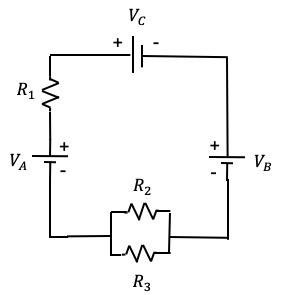The circuit shown in the figure below contains three resistors (R1, R2, and R3) and three batteries (VA, VB, and VC).  The resistor values are: R1=2 Ohms, R2=R3=6 Ohms, and the battery voltages are VA=25 V, VB=15 V, and VC=20 V.  When the circuit is connected, what will be the power dissipated by R2? VC HA R1 + + VA VB R2 M R3

• ### The circuit shown in the figure below contains three resistors (R1, R2, and R3) and three...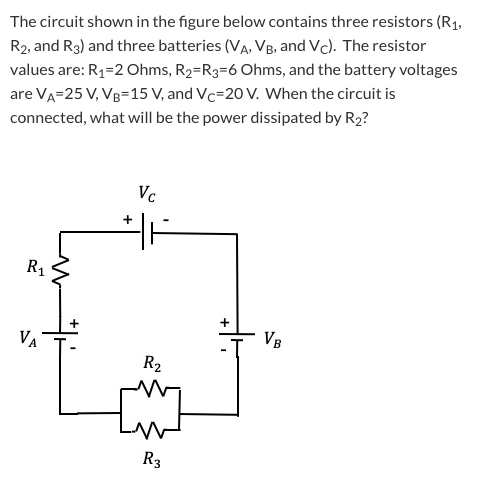The circuit shown in the figure below contains three resistors (R1, R2, and R3) and three batteries (VĄ, VB, and Vc). The resistor values are: R1=2 Ohms, R2=R3=6 Ohms, and the battery voltages are VA=25 V, VB=15 V, and Vc=20 V. When the circuit is connected, what will be the power dissipated by R2? VC HA R1 + VA + VB R2 my R3

• ### The circuit shown in the figure below contains three resistors (R1, R2, and R3) and three...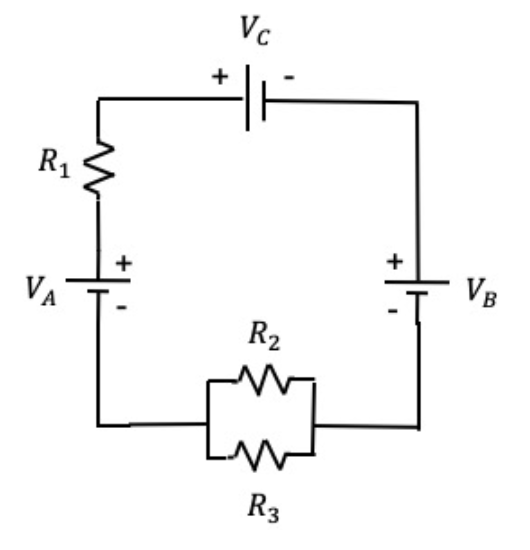The circuit shown in the figure below contains three resistors (R1, R2, and R3) and three batteries (VA, VB, and VC). The resistor values are: R1=2 Ohms, R2=R3=4 Ohms, and the battery voltages are VA=25 V, VB=15 V, and VC=20 V. When the circuit is connected, what will be the power dissipated by R3? options: 1.25 W 2.0 W 5.0 W 6.25 W 8.13 W Vc + R1 + + VA VB R2 R3